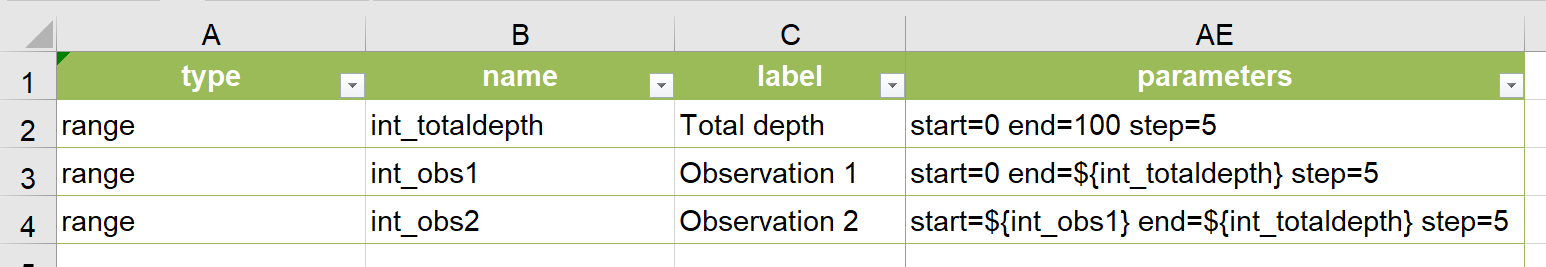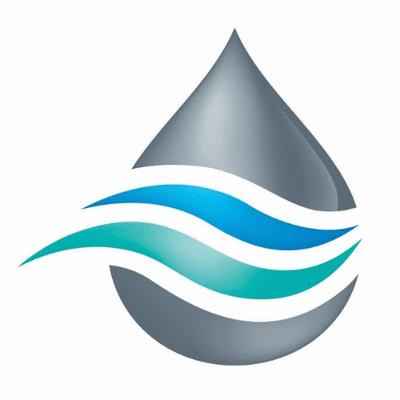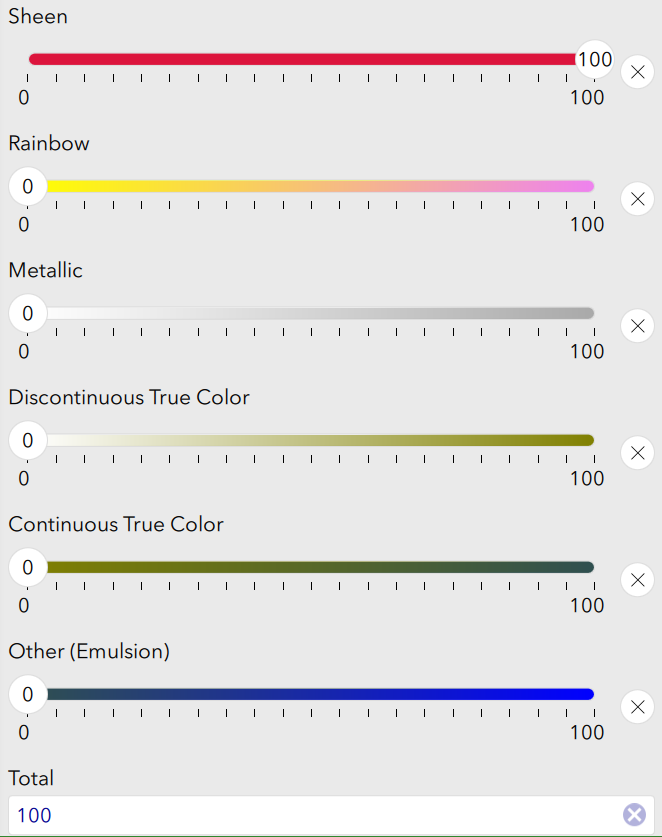# Dynamic min, max & step values for Survey123 range questions

1451
1
04-30-2020 01:14 AM
Status: OpenNew Contributor II

I would like to be able to dynamically set properties for a range question in Survey123.

Consider the following example: I have a slider used to specify the total depth of a sample (int_totaldepth) and other sliders used to specify the depths of observations along the core (int_obs1,int_obs2). In this case I would like to dynamically alter the parameters of "int_obs1" so that the maximum value is equal to "int_totaldepth". Something like this: "start=0 end=\${int_totaldepth} step=1". For the second observation slider I would like to set the ranges as follows: "start=\${int_obs1} end=\${int_totaldepth} step=1". These statements currently result in an error stating that the parameters must all be numbers.

It isn't relevant for my purpose, but in some circumstances it could be useful to be able to dynamically change the step value of a range question. For example the resolution of each step could be changed based on a previously selected method of measurement.

I realise that input can be controlled using constraints however I would like to provide the users with an interface that only presents viable options.Tags (3)
1 CommentI also have this request.

To add another use case.  We want to split an observed area of oil into different categories summing up to 100%.  As you see the below with Sheen occupying 100% all the other values must equal 0.  If Sheen was 50% then that would leave 50% to split over the other categories.

We've also used constraints to restrict incorrect answers but using dynamic variables will improve the users experience.